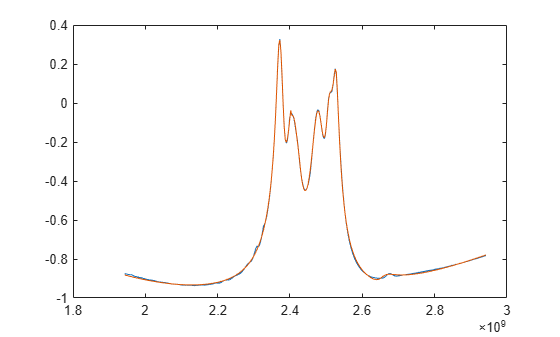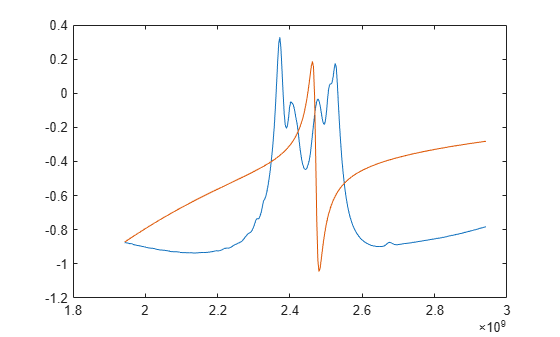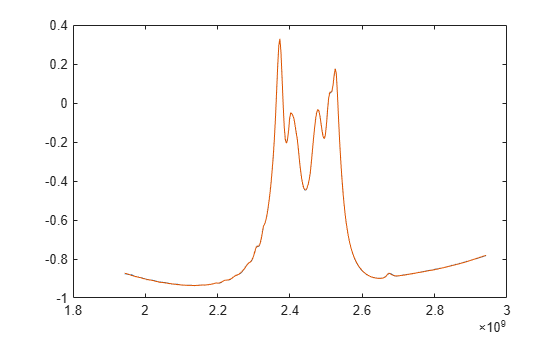# Using Rational Object to Fit S-Parameters

This example shows how to use the rational object to create a rational fit to S-parameter data, and the various properties and methods that are included in the rational object.

### Create rational object

Read in the sparameters, and create the rational object from them. The rational function automatically fits all entries of the S-parameter matrices.

`S = sparameters('sawfilter.s2p')`
```S = sparameters: S-parameters object NumPorts: 2 Frequencies: [334x1 double] Parameters: [2x2x334 double] Impedance: 50 rfparam(obj,i,j) returns S-parameter Sij ```
`r = rational(S)`
```r = rational with properties: NumPorts: 2 NumPoles: 35 Poles: [35x1 double] Residues: [2x2x35 double] DirectTerm: [2x2 double] ErrDB: -44.8394 ```

With the default settings on this example, the rational function achieves an accuracy of about -26 dB, using 30 poles. By construction, the rational object is causal, with a non-zero direct term.

### Compare fit with original data

Generate the frequency response from the rational object, and compare one of the entries with the original data.

```resp = freqresp(r, S.Frequencies); plot(S.Frequencies, real(rfparam(S, 1, 1)), ... S.Frequencies, real(squeeze(resp(1,1,:))))```### Limit number of poles

Redo the fit, limiting the number of poles to a maximum of 5. The rational object may use fewer poles than specified. Notice that the quality of the fit is degraded as opposed to the original 30-pole fit.

`r5 = rational(S, 'MaxPoles', 5)`
```r5 = rational with properties: NumPorts: 2 NumPoles: 4 Poles: [4x1 double] Residues: [2x2x4 double] DirectTerm: [2x2 double] ErrDB: -1.2663 ```
```resp5 = freqresp(r5, S.Frequencies); plot(S.Frequencies, real(rfparam(S, 1, 1)), ... S.Frequencies, real(squeeze(resp5(1,1,:))))```### Tighten target accuracy

Redo the fit, asking for a tighter tolerance (-60dB), Notice that the fit is significantly improved, particularly in the stopbands of the sawfilter.

`rgood = rational(S, -60)`
```rgood = rational with properties: NumPorts: 2 NumPoles: 148 Poles: [148x1 double] Residues: [2x2x148 double] DirectTerm: [2x2 double] ErrDB: -53.8055 ```
```respgood = freqresp(rgood, S.Frequencies); plot(S.Frequencies, real(rfparam(S, 1, 1)), ... S.Frequencies, real(squeeze(respgood(1,1,:))))```